﻿ Empirical Evaluation of Slag Cement Minimum Setting Time (SCMST) by Optimization of Gypsum Addition to Foundry Slag during Production

### Empirical Evaluation of Slag Cement Minimum Setting Time (SCMST) by Optimization of Gypsum Addition ...

C. I. Nwoye, I. Obuekwe, C. N. Mbah, S. E. Ede, C. C. Nwangwu, D. D. AbubakarOPEN ACCESSPEER-REVIEWED

## Empirical Evaluation of Slag Cement Minimum Setting Time (SCMST) by Optimization of Gypsum Addition to Foundry Slag during Production

C. I. Nwoye1,, I. Obuekwe1, 2, C. N. Mbah3, S. E. Ede3, C. C. Nwangwu1, D. D. Abubakar1, 4

1Department of Metallurgical and Materials Engineering, Nnamdi Azikiwe University, Awka, Nigeria

2Scientific Equipment Development Institute, Enugu, Nigeria

3Department of Metallurgical and Materials Engineering, Enugu State University of Science & Technology, Enugu, Nigeria

4Ajaokuta Steel Company, Kogi State, Nigeria

### Abstract

An empirical evaluation of slag cement minimum setting time (SCMST) has been successfully carried out through optimization of gypsum addition to foundry slag in the course of the cement production. A model was derived and used as a tool for predictive analysis of the cement setting time based on gypsum input. The model aided optimization of gypsum addition indicates a minimum setting time of 14.1054 minutes at an optimum gypsum input concentration of 6.4847%. Beyond 6.4847% gypsum addition, the slag cement setting time increases drastically; a situation typifying immiscibility and lack of homogeneity between the cement slurry and the extra gypsum addition. This is because increased gypsum addition (above a specified quantity) is unlikely to forms a coherent mass with a specified and fixed liquid volume, resulting to delayed and differential setting. The derived model expressed as;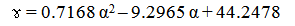is quadratic and single factorial in nature. The slag cement setting time per unit gypsum addition are as obtained from experiment and derived model are 4.75 and 4.996 mins./% respectively. Statistical analysis of the experimental and derived model-predicted results for each value of the gypsum input concentration considered shows standard errors of 1.8974 and 1.5485% respectively. Deviational analysis indicates 10.46% as the maximum deviation of the model-predicted slag cement setting time from the corresponding experimental value. The validity of the model was rooted on the expression 0.0226 ɤ + 0.2101 α = 0.0162 α2 + 1.0001 where both sides of the expression are correspondingly approximately equal. The validity of the derived model-predicted results also was ascertained using SPSS 17.0. The results indicate check variance = 0.001, standard deviation = 0, and model operational confidence of 95.0% at a significant level: 0.05.

### At a glance: Figures

123
Prev Next

• Nwoye, C. I., et al. "Empirical Evaluation of Slag Cement Minimum Setting Time (SCMST) by Optimization of Gypsum Addition to Foundry Slag during Production." American Journal of Mining and Metallurgy 2.3 (2014): 51-56.
• Nwoye, C. I. , Obuekwe, I. , Mbah, C. N. , Ede, S. E. , Nwangwu, C. C. , & Abubakar, D. D. (2014). Empirical Evaluation of Slag Cement Minimum Setting Time (SCMST) by Optimization of Gypsum Addition to Foundry Slag during Production. American Journal of Mining and Metallurgy, 2(3), 51-56.
• Nwoye, C. I., I. Obuekwe, C. N. Mbah, S. E. Ede, C. C. Nwangwu, and D. D. Abubakar. "Empirical Evaluation of Slag Cement Minimum Setting Time (SCMST) by Optimization of Gypsum Addition to Foundry Slag during Production." American Journal of Mining and Metallurgy 2, no. 3 (2014): 51-56.

 Import into BibTeX Import into EndNote Import into RefMan Import into RefWorks

### 1. Introduction

The growing need to support research and development aimed at improving the quality of cement produced through incorporation of industrial wastes and cheaper locally acquired material stems on the fact that cement plays a very important and unavoidable role in the building and construction sector. There is also need to evaluate other materials to produce materials of similar characteristics to cement.

The need to satisfy specification on application of cement has influenced the addition of materials in the course of cement production that would have the necessary performance characteristics . As it concerns health and environment, it should be inoffensive. Finally, the incorporation of the waste should not impair concrete durability. Traditional assessment methods must therefore be adopted to evaluate these new materials . The high content of soluble salt (Sugar) in ash has identified it as experimental waste . The environmental impact and durability of concrete are closely connected to its transport properties, which control the kinetics of the penetration of water and aggressive agents into concrete . Concrete diffusivity is practically linked to the movement of chemical species within the material and the leaching of certain chemicals .

The essential components of blast furnace slag has been discovered  to be of same oxide (such as lime, silica, and aluminum) as are present in Portland cement, but differ in proportion. Blast furnace slag is a by-product obtained in the manufacture of pig iron in the blast furnace. It has found application in the road and building industries and in the production of cementing materials, as an aggregate in concrete and in the manufacture of slag wool for thermal insulation.

The aim of this work is to evaluate empirically the slag cement minimum setting time (SCMST) through optimization of gypsum addition to foundry slag in the course of the cement production. A model would be derived and used as a tool for predictive analysis of the cement setting time based on gypsum input.

### 2. Materials and Methods

Materials used and their respective sources and details of the experimental procedure and associated process conditions are as stated in the past report .

2.1. Model Formulation

Experimental data obtained from research work  were used for this work. Computational analysis of the experimental data  shown in Table 1, gave rise to Table 2 which indicate that;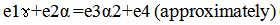(1)

Introducing the values of the empirical constants e1, e2, e3 and e4 into equation (1) reduces it to;(2)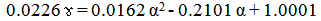(3)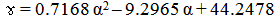(4)

Where

(ɤ) = Setting time (mins.)

e1 = 0.0226; Empirical constant (determined using C-NIKBRAN 

(α) = Concentration of gypsum added (%)

e2 = 0.2101; Empirical constant (determined using C- NIKBRAN )

e3 = 0.0162; Empirical constant (determined using C-NIKBRAN )

e4= 1.0001; Slag grade (determined using C-NIKBRAN )

During the model development, a soft ware; C-NIKBRAN was used to map-link parameters such setting time ɤ, and concentration of gypsum addition α, with the view to establishing an empirical relationship between ɤ and α. Map-linking was carried out using experimental results in Table 1. The result of the data mapping is the core model expression in equation (2), which on evaluation gives Table 2.

### 3. Boundary and Initial Condition

Consider foundry slag paste of required consistency (in a cylindrical container) interacting with quantities of gypsum. The container’s atmosphere was not contaminated i.e (free of unwanted gases, dusts and other micro organisms). Range of gypsum addition: 1-5%. Range of setting time considered (based on added gypsum): 16 - 35 mins., mass of wastes used, resident time, treatment temperature, and other process conditions are as stated in the experimental technique .

The boundary conditions are: free movement of oxygen across the cylindrical container. At the bottom of the particles, a zero gradient for the gas scalar are assumed and also for the gas phase at the top of the slag paste. The treated slag paste was stationary. The sides of the waste particles are taken to be symmetries.

### 4. Results and Discussion

The derived model is equation (4). The computational analysis of Table 1 gave rise to Table 2.

#### Table 2. Variation of 0.0226 ɤ + 0.2101α with 0.0162α2 + 1.0001

4.1. Model Validation

The validity of the model is strongly rooted on equation (2) where both sides of the equation are correspondingly approximately equal to 1. Table 2 also agrees with equation (2) following the values of 0.0226 ɤ + 0.2101α and 0.0162 α2 + 1.0001 evaluated from the experimental results in Table 1. Furthermore, the derived model was validated by comparing the setting time (of the slag cement) predicted by the model and that obtained from the experiment . This was done using the 4th Degree Model Validity Test Techniques (4th DMVTT); computational, graphical, statistical and deviational analysis .

The empirical model derived for evaluating the slag cement minimum setting time ɤ relates ɤ with α such that slag cement minimum setting time could be determined (as model out-put) at any point in time without further experimental work by substituting the highlighted input parameter α (concentration of gypsum addition) into the derived model, providing the input parameter is within the range of values generated during the initial experimental work under the initial experimental conditions (Boundary and initial conditions).

Based on the forgoing, substitution of the model input parameter α into the derived model, outside these boundary and initial conditions: gypsum addition: 1-5%, setting time considered: (based on gypsum addition): 16 - 35 mins, makes the predicted slag cement minimum setting time unreliable. The initial condition is rooted on the condition that the foundry slag should be in paste form and the atmosphere inherent in the processing container must not be contaminated i.e (free of unwanted gases, dusts and other micro organisms).

The limitation of the derived model is that it cannot operate outside the highlighted boundary and initial conditions of the initial experimental work. The derived model could be improved on by adding a correction factor to the derived model expression so as to take care of the prevailing experimental conditions, which play vital roles during the experiment, but were not considered during model formulation.

4.1.1. Computational Analysis

A critical comparative analysis of the results computed from experimental and model-predicted setting time was carried out to ascertain the degree of validity of the derived model. This was done by comparing setting time per unit gypsum addition obtained by calculations involving experimental results, and model-predicted results obtained directly from the model.

Figure 1. Coefficient of determination between setting time and concentration of gypsum added as obtained from the experiment

Setting time of slag cement per unit gypsum addition ɤg (mins./%) was calculated from the equation;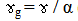(5)

Therefore, a plot of setting time against concentration of gypsum addition as in Figure 1 using experimental results in Table 1, gives a slope, S at points (1, 35) and (5, 16) following their substitution into the mathematical expression;(6)

Equation (6) is detailed as(7)

Where

Δɤ = Change in the setting time ɤ2, ɤ1 at two gypsum concentrations α2, α 1. Considering the points (1, 35) and (5, 16) for (α1, ɤ1) and (α2, ɤ 2) respectively, and substituting them into equation (7), gives the slope as 4.75 mins./% which is the slag cement setting time per unit gypsum addition during the actual experimental process. Also similar plot (as in Figure 2) using model-predicted results gives a slope. Considering points (1, 35.6681) and (5, 15.6853) for (α1, ɤ1) and (α2, ɤ2) respectively and substituting them into equation (7) gives the value of slope, S as 4.996 mins./%. This is the model-predicted slag cement setting time per unit gypsum addition. A comparison of these two values of the slag cement setting time per unit gypsum addition shows proximate agreement.

Figure 2. Coefficient of determination between setting time and concentration of gypsum input as predicted by derived model
4.2. Statistical Analysis
4.2.1. Standard Error (STEYX)

The standard errors (STEYX) in predicting the setting time (using results from derived model, and experiment) for each value of the concentration of added gypsum are 1.5485 and 1.8974% respectively. The standard error was evaluated using Microsoft Excel version 2003.

4.2.2. Correlation (CORREL)

The correlations between setting time and gypsum input concentration as obtained from derived model and experiment considering the coefficient of determination R2 from Figure 1 and Figure 2 was calculated using Microsoft Excel version 2003.(8)

The evaluations correlations are shown in Table 3. These evaluated results indicate that the derived model predictions are significantly reliable and hence valid considering its proximate agreement with results from actual experiment.

#### Table 3. Comparison of the correlations evaluated from D-Model predicted and ExD results based on gypsum input concentration

4.3. Graphical Analysis

Comparative graphical analysis of Figure 3 shows very close alignment of the curves from the experimental (ExD) and model-predicted (MoD) slag cement setting time relative to gypsum addition respectively. Furthermore, the degree of alignment of these curves is indicative of the proximate agreement between both experimental and model-predicted setting time.

Figure 3. Comparison of the setting times relative to concentration of gypsum input as obtained from experiment and derived model.
4.4. Comparison of Derived Model with Standard Model
Figure 4. Comparison of the setting time relative to concentration of gypsum input as obtained from experiment (ExD) , derived model (MoD) and regression model (Reg).
Figure 5. Coefficient of determination between setting time and concentration of gypsum added as predicted by regression model

The validity of the derived model was further verified through application of the regression model (Reg) (Least Square Method using Excel version 2003) in predicting the trend of the experimental results. Comparative analysis of Figure 3 and Figure 4 shows very close alignment of curves and significantly similar trend of data point’s distribution for experimental (ExD) and derived model-predicted (MoD) results of setting time. Figure 5 shows that the calculated correlations between setting time and gypsum input concentration for results obtained from regression model gives 1.000. The slag cement setting time per unit gypsum addition as obtained from regression model is 5.0 mins./%. These values are in proximate agreement with both experimental and derived model-predicted results.

Results generated from experiment, derived model and regression model show that the slag cement setting time decreases with increase in the concentration of gypsum addition. The correlations between slag cement setting time and concentration of gypsum addition as obtained from experiment, derived model and regression model were all > 0.97.

4.5. Validation of Model-predicted Results Using SPSS 17.0

The validity of the derived model-predicted results also was ascertained using SPSS 17.0. The results indicate check variance = 0.001, standard deviation = 0, and model operational confidence of 95.0% at a significant level: 0.05.

4.6. Deviational Analysis

Comparative analysis of setting time from experiment  and derived model revealed insignificant deviations on the part of the model-predicted values relative to values obtained from the experiment. This is attributed to the fact that the surface properties of the slag material and the physiochemical interactions between the slag material and added gypsum (under the influence of the treatment temperature) which were found to have played vital roles during the process  were not considered during the model formulation. This necessitated the introduction of correction factor, to bring the model-predicted compressive strength to those of the corresponding experimental values.

Deviation (Dv) of model-predicted setting time from that of the experiment  is given by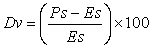(9)

Correction factor (C ) is the negative of the deviation i.e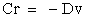(10)

Therefore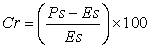(11)

Where

Ps = Model-predicted setting time ( mins.)

Es = Setting time obtained from experiment (mins.)

Cr = Correction factor (%)

Dv = Deviation (%)

Introduction of the corresponding values of Cr from equation (11) into the model gives exactly the corresponding experimental setting time.

Comparative analysis of Figure 6 shows that the maximum deviation of the model-predicted slag cement setting time from the corresponding experimental values is less than 11% and quite within the acceptable deviation limit of experimental results. This invariably translated into over 89% operational confidence for the derived model as well as over 0.89 dependency coefficients of slag cement setting time on gypsum addition.

These figures show that least and highest magnitudes of deviation of the model-predicted setting time (from the corresponding experimental values) are + 10.46 and – 2.32% which corresponds to setting times: 15.6853 and 28.522 mins./% as well as concentration of gypsum addition: 5 and 2% respectively.

Analysis of Figs. 6 and 7 indicates that the orientation of the curve in Figure 7 is opposite those of the deviation of model-predicted setting time in Figure 6. This is because correction factor is the negative of the deviation as shown in equations (10) and (11). It is believed that the correction factor takes care of the effects of the surface properties of the slag material and the physiochemical interaction between the slag material and the added gypsum which (affected experimental results) were not considered during the model formulation. Figure 7 indicate that the least and highest magnitudes of correction factor to the model-predicted compressive strength are – 10.46 and + 2.32% which corresponds to setting times: 15.6853 and 28.522 mins./% as well as concentration of gypsum addition: 5 and 2% respectively.

The setting time predicted by the derived model (equation (6)); ɤ = 0.7168 α2 – 9.2965 α + 44.2478 is based on the concentration of gypsum addition. Optimization of gypsum addition was achieved by differentiating the derived models (equations (6)) with respect to α (and equating to zero) in order to determine the value of α at which ɤ is minimum.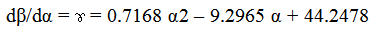(12)

Differentiation of equations (12) with respect to α reduces them respectively to;(13)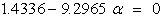(14)

The value of α = 6.4847%, evaluated from equations (14) is the optimum concentration of gypsum addition which invariably gives the minimum setting time, ɤ as 14.1054 mins. on substituting the value of α = 6.4847% into the derived model in equation (6).

Figure 8 shows that beyond 6.4847% gypsum addition, the slag cement setting time increases drastically; a situation typifying immiscibility and lack of homogeneity between the cement slurry and the extra gypsum addition. This is because increased gypsum addition (above a specified quantity) is unlikely to forms a coherent mass with a specified and fixed liquid volume, resulting to delayed and differential setting.

Figure 8. Predicted minimum setting time of the slag cement at predicted optimum concentration of gypsum addition

Confirmation of the minimum and optimum values of ɤ and α respectively was carried out by substituting an assumed range of values of the concentration of gypsum addition α = 8, 10, 12 and 15%. Figure 8 strongly shows that addition of gypsum below or above the optimum value 6.4847% would give untargeted and obscured result. This is because gypsum addition below the optimum value only lowers the cement setting time and above this optimum, cement setting time increases at the expense of quality transfer to other productions. For example, increase in the cement setting time might hamper some mechanical properties of concrete made with the cement. This is so because prolonged setting of the concrete could be negatively affect by natural constraints like rain fall which drops after periodic increase in the atmospheric relative humidity.

### 5. Conclusion

An empirical evaluation of slag cement minimum setting time (SCMST) was successfully carried out through optimization of gypsum addition to foundry slag in the course of the cement production. A model was derived and used as a tool for predictive analysis of the cement setting time based on gypsum input. The model aided optimization of gypsum addition indicated a minimum setting time of 14.1054 minutes at an optimum gypsum input concentration of 6.4847%. Beyond 6.4847% gypsum addition, the slag cement setting time increased drastically; a situation typifying immiscibility and lack of homogeneity between the cement slurry and the extra gypsum addition. This is because increased gypsum addition (above a specified quantity) is unlikely to forms a coherent mass with a specified and fixed liquid volume, resulting to delayed and differential setting. The slag cement setting time per unit gypsum addition are as obtained from experiment and derived model are 4.75 and 4.996 mins./% respectively. Statistical analysis of the experimental and derived model-predicted results for each value of the gypsum input concentration considered shows standard errors of 1.8974 and 1.5485% respectively. Deviational analysis indicates 10.46% as the maximum deviation of the model-predicted slag cement setting time from the corresponding experimental value. The validity of the model was rooted on the expression 0.0226 ɤ + 0.2101 α = 0.0162 α2 + 1 where both sides of the expression are correspondingly approximately equal. The validity of the derived model-predicted results also was ascertained using SPSS 17.0. The results indicate check variance = 0.001, standard deviation = 0, and model operational confidence of 95.0% at a significant level: 0.05.

### References

  Helmuth, R. (1987). Fly ash in cement and concrete, Portland Cement Association. Journal of Cement and Concrete Research, 30: 201-204In article  Chatterji, A. K. (1990). Adsorption of lime and pozzolanic activity. Journal of Scientific Industrial Research, 19B: 493-494.In article  Kessler, B., Rollet, M., and Sorrentino, F. (1992). Microstructure of cement pastes as incinerators ash host. Proceedings of 1st International Symposium on cement industry solution to waste Management, Calgary. Pp 235-251.In article  Pimienta, P., Remond, S., Rodrigues, N and Bournazel, J. P. (1999). Assessing the Properties of Mortars Containing Musical Solid Waste Incineration Fly Ash. International Congress, Creating with Concrete, University of Dundee. Pp 319-326.In article  Remond, S., Pimienta, P., and Bentz, D. P. (2002). Effect of the incorporation of municipal solid waste fly ash in cement paste and Mortars. Journal of cement and concrete research, 10:12-14In article  Egunlae, O. O. (2011). The Influence of Gypsum on Foundry Slag for Making Cement. Inter. Res. J. Eng. Sc. & Tech., 8 (1): 33-42In article  Nwoye, C. I. (2008). Data Analytical Memory; C-NIKBRANIn article  Nwoye, C. I. and J. T. Nwabanne. (2013). Empirical Analysis of Methane Gas Yield Dependence on Organic Loading Rate during Microbial Treatment of Fruit Wastes in Digester. Advances in Applied Science Research, 4 (1): 308-318.In article